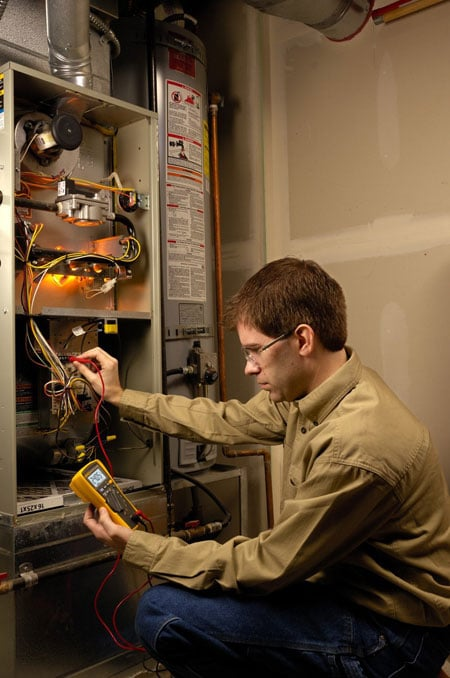Select Page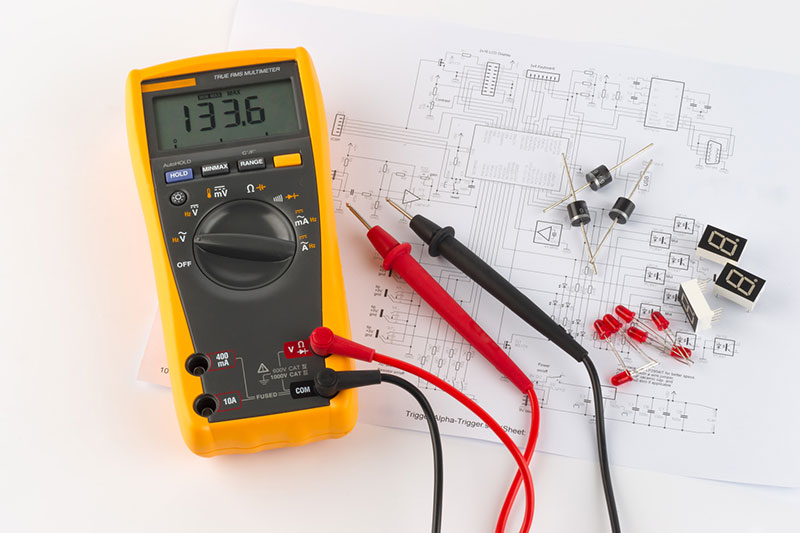A multimeter is a test tool used to measure two or more electrical values–principally current (amps), voltage (volts) and resistance (ohms) typically over several ranges of value.

In modern industrial plants, homes, and offices, measuring current accurately is more difficult than ever.

As we rely heavily on computers and other complex machinery that draws current in short pulses rather than at a steady level, the accuracy of a standard multimeter is compromised.

This means that the results you get from a digital multimeter may not be reliable in most cases. So what can you do to fix this?

The simple Solution is to invest in a true RMS multimeter.

When a multimeter is used to measure DC current, the result is accurate and straightforward. However, the same cannot be said for AC current.

This is because AC current travels back and forth down a conductor and can best be described in a graphical presentation. In simple terms, it is transmitted using a sine wave hence constantly changing in voltage.

For AC currents with a perfect sine wave, the RMS (root mean square) current will always be a constant factor (0.707) of the peak current.

However, perfect sine waves are rare in both industrial and commercial applications.

So, what happens when the current you are measuring is not perfect sine wave? The above rule no longer applies.

In such cases, use of standard multimeter generates unreliable results and only a true RMS multimeter can give you accurate results.

But if the AC current whose RMS value you want to measure is a pure sine wave, then you won’t need a true RMS multimeter.

## What is true RMS multimeter?

When current is non-linear, you’ll need a reliable meter that can measure the current with the highest level of accuracy.

There are two ways of doing this. You can either use a waveform capturing equipment to check the waveform or you can simply purchase a true RMS multimeter to get reliable results.

True RMS is an advanced measurement technique used by digital multimeters which provide a real, effective value of alternating current (AC) levels.

It doesn’t matter if the wave is linear or non-linear (perfect or distorted), a true RMS multimeter adjusts itself accordingly to deliver the accurate results you desire.

Practically it saves you time and effort and at the same time clears all confusion when testing.

If you are serious about investing in a reliable meter, a true RMS multimeter should take precedence on your shopping list.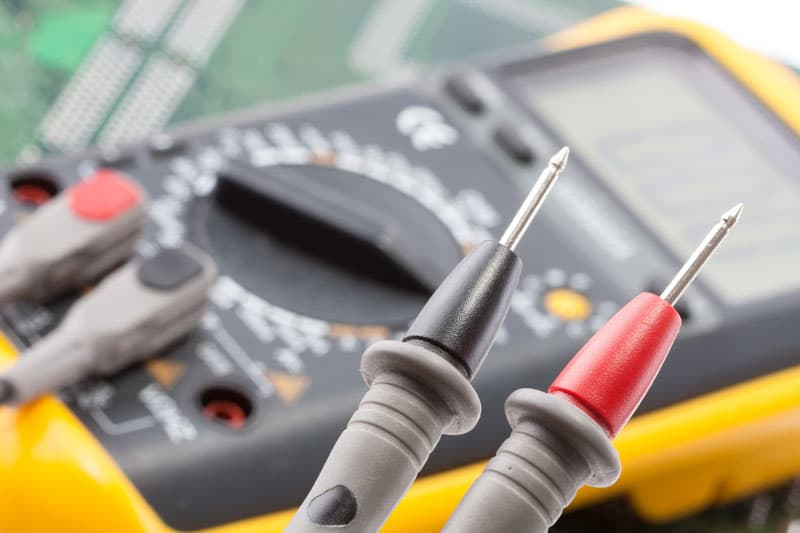## Why true RMS?

Before we get to understand why true RMS is the most preferred tool for measuring alternating current, let’s define the basics first.

• Sinusoidal waves (sine waves) – These are pure waves with no distortions, characterized by symmetrical transitions between peaks and valleys.
• Nonsinusoidal waves – Waves with irregular, or distorted patterns – squares, triangles, pulse trains, spikes, and other rugged and angular waves

RMS (root mean square) effectively calculates the equivalent DC (direct current) value of an AC waveform.

Though there are many ways for measuring alternating current, the most accurate way is the use of true RMS method. However, measuring RMS values is more complicated than it sounds.

Why bother if it’s that complicated?

Because true RMS is the only measurement that gives AC voltage readings that are independent of the shape of the signal.

An average meter uses basic mathematical formulas to measure pure sinusoidal waves. It can also be used to measure nonsinusoidal waves, but with a low accuracy (up to 40% low or 10% higher in its calculations)

A true RMS multimeter, on the other hand,  can measure both sinusoidal and nonsinusoidal waves with a higher degree of accuracy.

## RMS multimeter crest factor

When buying a true RMS multimeter, it’s important to check the RMS crest factor. This tells you how badly off the waveform is, and can be calculated by dividing the current’s peak value by the effective true RMS value.

The crest factor for a perfect sine wave is 1.414. The more the wave distorts the higher this value moves.

So if your RMS multimeter has a crest factor of 1.5, you will still obtain inaccurate results on waves above this threshold.

However, if you buy a digital multimeter with a crest factor of 3 or higher, you should obtain accurate results no matter the degree of distortion of the waveforms.

## Tips for better RMS measurements

Considering the importance and the difficulties encountered in measuring true RMS values, what should you do to achieve better results? The following tips will help you achieve more accurate results.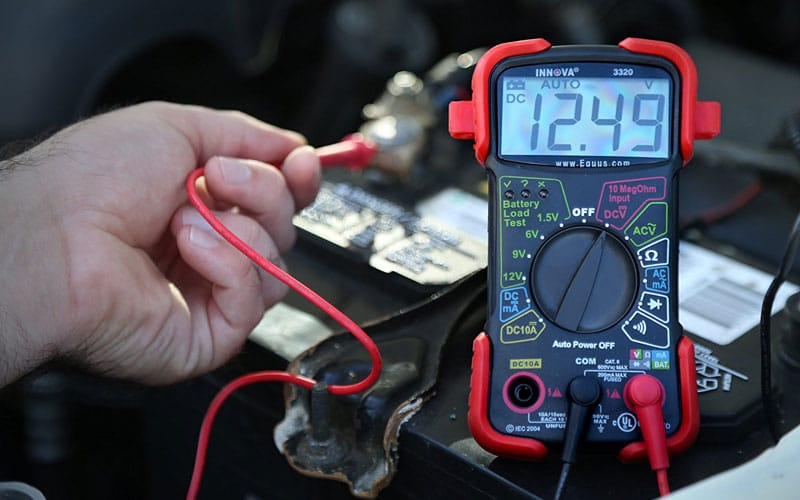## Tip 1: Understand how your digital multimeter measures RMS

When it comes to measuring RMS values, multimeters are not created equal. Understanding the technology that a multimeter uses to measure RMS can help you decide if it meets your needs.

The following is a summary of the four common multimeters technologies and their suitability for use.

### 1. Thermal AC to DC converters

This older technology of measuring RMS values is rarely used today. It employs the equivalent heating value approach, whereby the AC current heats a thermocouple while the meter’s DC section reads the thermocouple output.

One advantage of this technique is that it can accurately measure very high crest factors as well as handle high bandwidth measurements, so it can give true RMS values for a wide range of real-world signals.

On the downside, the thermal approach lacks the flexibility to trade off measurement speed with low-frequency accuracy.

If high accuracy is required and you are measuring high crest factor signals, seeking out one of these thermal models can get you accurate results.

### 2.  Analog AC to DC converters

Most high-end and mid-range digital multimeters use a chain of analog circuits to calculate the RMS values of the AC signal. The converter circuitry goes through a process to compute the RMS values.

First, it squares the input signal. Next, it passes the input signal through a circuit that averages the signal. Lastly, it runs through circuitry that computes the square root of the signal.

The result is an RMS value that is independent of the waveform being applied.

These inexpensive meters generates fast and accurate results. However, they do have some limitations regarding crest factor, bandwidth, and accuracy levels. Be sure to check more of this on your multimeters data sheet.

### 3. Digital sampling AC converters

Some digital multimeters use digital sampling techniques, which is similar to the technique used in modern oscilloscopes to create a series of data points that are sent through an RMS algorithm.

Digital sampling uses multiple passes to capture a signal and with enough passes, the signal can be digitized with a very high resolution.

Digital sampling technique has many advantages over analog converters. For example, you can make better measurements of pulse trains and other low-frequency cycle signals.

It can also measure true RMS fast with high accuracy and in a wide variety of signals.

If high accuracy is of paramount importance to you, then you should consider buying a digital sampling RMS multimeter.

### 4. Peak and averaging AC converters

These inexpensive meters usually compute RMS levels from either average or peak values.

The true RMS values delivered are only for pure, undistorted sine waves.

These meters are not a viable option if the required measurements are for real-world signals.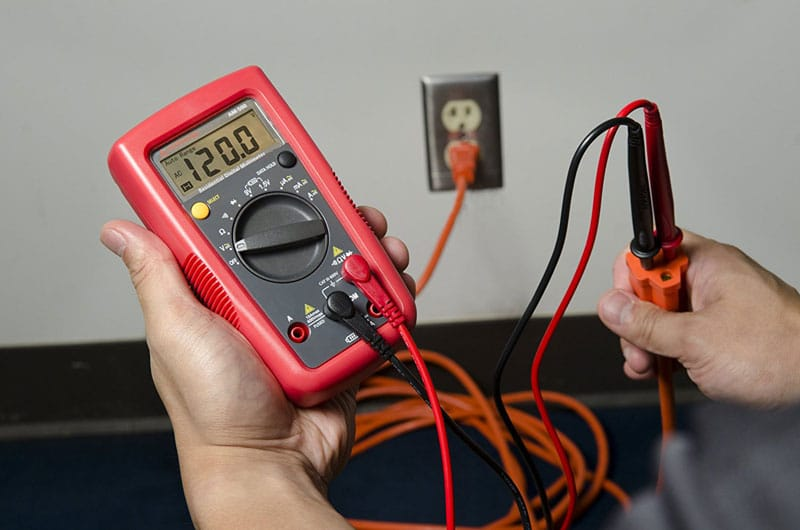## Tip 2: Avoid these common measurement errors

To make accurate measurements with your RMS multimeter, you’ll need to keep an eye on these factors that may lower the accuracy of your readings.

### 1. Harmonic content

Signals that are rich in harmonics can generate low reading measurements if less of these components are included in the measurements.

It’s also possible to get higher than actual values if only the fundamental is measured.

To avoid these errors, check your multimeter’s data sheet to know how much bandwidth you should work with.

### 2. Low-level measurement errors

When measuring AC voltages less than 100 mV, keep in mind that these measurements are susceptible to errors resulting from extraneous noise sources.

An exposed test lead can serve as an antenna, and your multimeter will measure these unwanted signals as well.

To eliminate or minimize these errors, use good shielding techniques, reduce the area of the antenna, and ensure both the AC source and the multimeter are connected to the same electrical outlet so as to reduce ground loops.

Most digital multimeters have a large DC blocking capacitor in their input path.

To improve your first reading accuracy, you’ll need to give the capacitor enough time to charge, especially when measuring low-frequency signals.

### 4. Setting time

RMS measurements require averaging of time over multiple periods of the lowest frequency being measured.

Make sure to select the appropriate low-frequency filter on your RMS multimeter to allow the fundamental to be captured.

The lower the AC filter frequency you set, the longer the settling time and hence the longer the time it will take to make measurements.

### 5. Measurements below full scale

Many digital multimeters specify AC inputs down to 5 or 10% of the full scale.

For better accuracy, measure as close to full scale as possible.

In some cases, you might need to override auto scaling. However, care should be taken when measuring high crest factor signals as overloading may saturate the meter’s input circuitry.

## Conclusion

An RMS multimeter is an indispensable component in your electrical toolkit.

It not only gives you electrical measurements with accuracy but also saves you a lot of time and money in the long run.

The need for true RMS multimeters has grown rapidly in the past few years as the possibility of nonsinusoidal waves in circuits keeps on growing.

In addition, a true RMS multimeter can also be very useful when measuring current where AC characteristics are unknown.